Introduction to Electronic Circuits: 2 of 3

In our previous session we reviewed the most common passive elements used in electronic circuits. This time we look at semiconductors, and at some of the important active elements made from them: diodes, transistors, and FETs.

## Semiconductor Between Conductors and Insulators

Semiconductors are positioned midway between conductors and insulators. They are the materials―such as silicon (Si) and germanium (Ge)―that resist electric current better than metals like silver and aluminum, but not as well as insulators like quartz and ceramic.

The resistivity of a material is dependent on the density of free electrons that can move readily when a voltage is applied. In general, this density can be engineered to any desired value by adding the appropriate impurities to a uniform material. This makes it possible to create semiconductors that provide just the right levels of conductivity for the purpose at hand.

A semiconductor may be either N-type or P-type, in accordance with the way current flows through it.

### How Current Moves Through a Semiconductor

#### (1) N-Type Semiconductors

Figure 1 illustrates a typical structure for an N-type semiconductor. This semiconductor consists of a silicon crystal that has been doped with atoms of phosphorus (P). A phosphorus atom has five valence electrons, four of which form strong covalent bonds with the adjacent silicon atoms, while the fifth remains free. At room temperatures, free electrons will often change places with nearby bound electrons; or, to put it another way, any given electron will sometimes be bound, and sometimes free. The result is that there is always a supply of free, mobile electrons available to carry a current. This kind of semiconductor is called "N-type" because it principally uses its (negatively charged) electrons to carry the current. Meanwhile, the donor (dopant) atoms that give up an electron to this process acquire positive charge.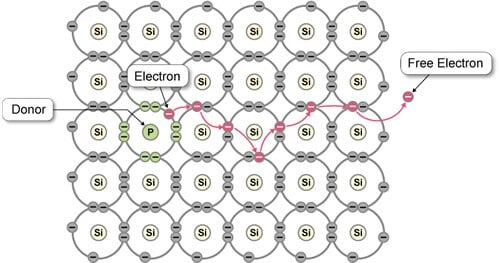Figure 1: Structure of an N-type Semiconductor

#### (2) P-Type Semiconductors

Figure 2 illustrates the structure of a P-type semiconductor. In this case, silicon crystal has been doped with atoms of boron (B). Again, each silicon atom has four valence electrons; but the boron atoms, with only three, are "deficient." Only a small amount of kinetic energy is needed to free an electron from an adjacent silicon atom; this electron is then quickly "accepted" by the boron atom. The silicon atom, however, is now left with a "hole" that soon pulls in an electron from another nearby silicon atom. As the process continues, the holes "move," producing a current. This kind of semiconductor is called "P-type," as most of its current is carried by holes. The dopant atoms that accept an electron in this process become negatively charged.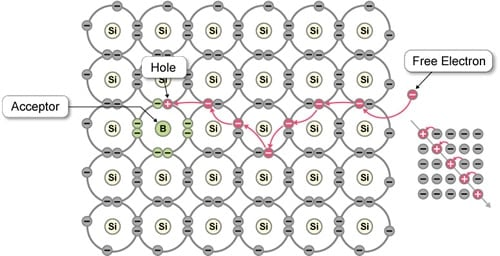Figure 2: Structure of a P-type Semiconductor

## Diodes: One-Way Streets

A semiconductor diode consists of a P-type semiconductor on one side and an N-type on the other. The carriers from each side diffuse across the center area (the P-N junction) and pair off, creating a central region where no carriers exist. The electrically charged impurities in this area form an electrical boundary that stops further diffusion and pairing. This carrier-free boundary region is called the depletion layer.

If a positive voltage is applied to the terminal at the P-type end of the diode, and a negative voltage to the terminal at the N-type side, this "forward" voltage will push more carriers (holes on P-type side, electrons on the N-type side) into the depletion layer, making it narrower, and causing more carriers to diffuse over the boundary, pair off, and disappear. Meanwhile, the applied voltage generates a current that continues to supply more carriers, so that the current can keep flowing.

If a reverse-bias voltage is applied, however (negative on the P-side, positive on the N-type side), then this voltage will pull carriers away from the center and toward the respective terminals, widening the depletion layer so that current flow is blocked.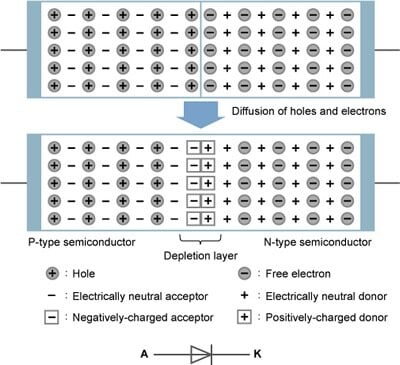Figure 3: Structure of a PN-Junction Diode

The result is that the diode passes current in only one direction (called the forward direction), and blocks current from moving the other way (the reverse direction). A diode used in this way is also called a rectifier: a component that passes current in one direction only.

## Voltage and Current Characteristic of a Diode

Figure 4 shows the electrical characteristics of a diode. Note that there will be no current at all―not even in the forward direction―if no voltage is applied. The voltage required to start current flow in the forward direction varies according to the semiconductor material: 0.7 to 0.8 V for a silicon diode, about 0.2 V for a Schottky barrier diode, and 2 to 5 V or more for a light-emitting diode (LED).

Considerably higher voltage would be required to get the current flowing the other way; the term breakdown voltage refers to the reverse voltage above which the current increases very rapidly as voltage goes higher. Because this breakdown voltage is essentially independent of current, these reverse-voltage characteristics can be used to implement voltage regulators and other such functionalities.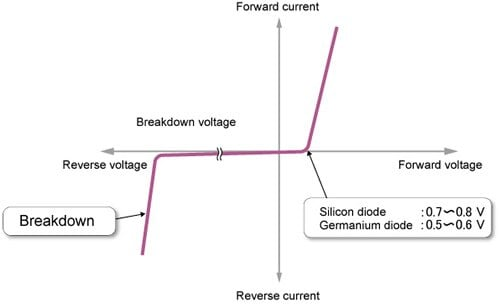Figure 4: Voltage and Current Characteristics of a Diode

## Solid-State Transistor: A Core Component First Started in Use

A bipolar transistor (as distinct from a field-effect transistor, described later) consists of a P-type or N-type center sandwiched between N-type or P-type exteriors. There are two main types: NPN-type (with the P-type at the center) and PNP-type (with an N-type at the center).

Figure 5 illustrates the operation of an NPN transistor. The base and emitter, taken together, have the same structure as a diode. In this example, application of a forward voltage (of about 0.7 V) causes base current (IB) to flow, so that many free electrons move from the emitter region into the base region. If the emitter is pumping out more carriers than can be recombined in the base region, the excess free electrons move into the collector area in accordance with applied voltage E2. In a typical application, the number of free electrons coming from the emitter will be 10 to 100 times the number that can recombine in the base region. The collector current (IC) will therefore exceed IB by the same factor (10 to 100). If IB is 0, then IC will also be 0, as the emitter will not be releasing any carriers.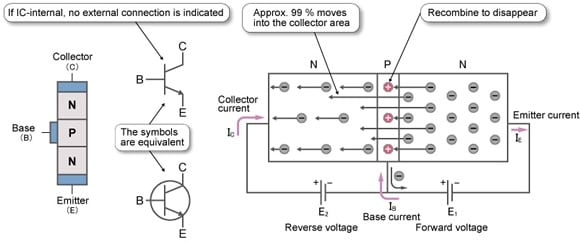Figure 5: NPN Transistor

In other words, the forward current IB between the base and emitter effectively controls current IC between the emitter and the collector. Thanks to this feature, transistors can be used alone to implement switches and amplifiers, while they are also the most essential component in modern electronic circuitry. They can be combined in many different ways to create many complex circuits.

## Transistors as Switches

As explained above, a transistor can produce a collector current that is many times greater than its base current. The ratio of collector current to base current is referred to as the forward current gain, or hFE. This gain can take a value between 100 and 700.

As we can see from the circuit shown in Figure 6 application of 0 V at the IN terminal results in zero base current, and therefore zero collector current. Accordingly, there is no current through load resistance RL, and the output at the OUT terminal is 12 V.

Now, if we apply a sufficiently high voltage between the base and emitter (generally about 0.7 V or higher vs. 0 V), this will cause base current to flow, which in turn will produce a collector current that is hFE times the base current; except that the actual current will be limited by resistance load RL to a value of ((12 V -- Vce-sat (saturation voltage)) / RL). This type of switching circuit is often utilized to enable a microcontroller, logic IC, or similar small component to drive a current-hungry device that it cannot drive directly―such as a power LED, a relay, or a DC motor.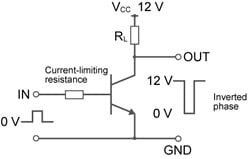Figure 6: Transistor Operating as a Switch

## Key Component of Integrated Circuits

There are two main types of FETs (field-effect transistors): MOSFETs (metal- oxide-semiconductor FETs) and JFETS (junction FETs). MOSFETs, in particular, have a flatter structure than the bipolar transistors discussed above, can be placed closer together without suffering from cross-interference, and can operate at low power. They therefore support better integration and miniaturization, and have become essential components of ICs and LSIs. Let's take a brief look at how a MOSFET works.

Figure 7 shows an N-type MOSFET. It has a gate terminal ("G"), under which is an oxide film that serves as an insulator. There is also a source terminal ("S") to one side of G, and a drain terminal ("D") to the other side. When there no voltage between the gate and source, the P-type semiconductor separating the source and the drain acts as an insulator. So current does not flow between the source and the drain.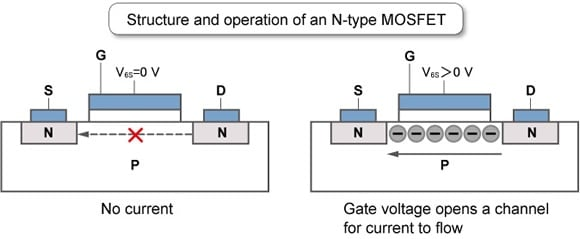Figure 7: N-type MOSFET

When a voltage is applied at the gate, however, it attracts and pulls up free electrons that are just under the gate, resulting in an abundance of these electrons in the area between the source and the drain. These electrons form a channel over which current can now flow freely.

In other words, the gate voltage is used to control the current flow between the source and the drain. MOSFETs are mainly used in switch circuits and amplifier circuits. They can also be used to provide a steady current, since application of a fixed voltage at the gate will generate a fixed current flow between source and drain.

On an N-type MOSFET, the channel is N-type. On a P-type MOSFET, the channel is P- type.

## CMOS Semiconductors: An Essential Element of Digital Circuits

A CMOS (complementary MOS) semiconductor is a set of MOSFETs connected as shown in Figure 8. When a voltage of either 0 V or VCC is applied at the IN terminal, one or the other of these MOSFETs will be on. This means that there is almost no current flow between VCC and GND. CMOS devices are therefore good for creating low-power logic circuits. Most modern LSIs and ICs are built with CMOS technology.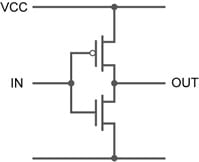Figure 8: CMOS Inverter

In our next session, we will learn about basic ICs and op-amps used to amplify analog signals.

## Module List

1. Passive Elements
2. Diodes, Transistors, and FETs
3. Op-Amps, Comparator Circuit